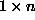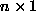Data Structures and Algorithms with Object-Oriented Design Patterns in C## Projects

1. Complete the implementation of the DynamicArray class given in Programto Program. Write a test suite to verify all of the functionality. Try to exercise every line of code in the implementation.
2. Complete the implementation of the LinkedList class given in Programto Program. Write a test suite to verify all of the functionality. Try to exercise every line of code in the implementation.
3. Change the implementation of the LinkedList class given in Programto Programby removing the tail field. That is, implement the singly-linked list variant shown in Figure(a). Write a test suite to verify all of the functionality. Try to exercise every line of code in the implementation.
4. Change the implementation of the LinkedList class given in Programto Programso that it uses a circular, singly-linked list with a sentinel as shown in Figure(c). Write a test suite to verify all of the functionality. Try to exercise every line of code in the implementation.
5. The MultiDimensionalArray class given in Programto Programonly supports subscript ranges starting at zero. Modify the implementation to allow an arbitrary subscript base in each dimension.
6. Design and implement a three-dimensional matrix class Matrix3D based on the two-dimensional class DenseMatrix given in Programto Program7. A row vector is amatrix and a column vector is anmatrix. Define and implement classes RowVector and ColumnVector as classes derived from the base class DynamicArray given in Programto Program. Show how these classes can be combined to implement the Matrix interface declared in Program.Copyright © 2001 by Bruno R. Preiss, P.Eng. All rights reserved.